# Java Program to Compare Two Arrays

In this tutorial you will learn about the Java Program to Compare Two Arrays and its application with practical example.

## Java Program to Compare Two Arrays

In this tutorial, we will learn to create a Java program that will Compare Two Arrays using Java programming.

## Prerequisites

Before starting with this tutorial, we assume that you are the best aware of the following Java programming topics:

• Operators in Java Programming.
• Basic Input and Output function in Java Programming.
• Basic Java programming.
• For loop in Java programming.
• Arithmetic operators in Java Programming.

## What is Compare?

In comparing, we compare two or more things point to point and find the common things or the differences in them.

## Compare Two Arrays.

In this program, First, we will declare arrays one and two for the program. Then we will compare them from each other. Then, if they satisfy the conditional statements, they will print that the arrays are the same.  If not then satisfy the conditional statements then will print that the arrays are not the same. With the help of this program, we can Compare Two Arrays in an Array.

## Output:-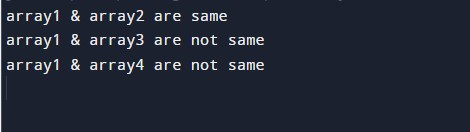In the above program, we have first initialized the required variable.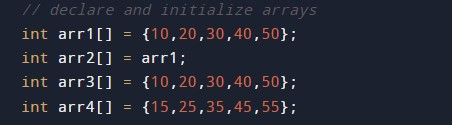• arr1  = it will hold the integer array.
• arr2  = it will hold the integer array.
• arr3  = it will hold the integer array.
• arr4  = it will hold the integer array.

Condition to check for comparison.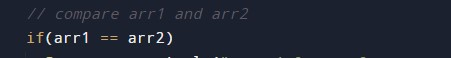Printing if the arrays are the same.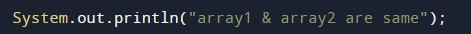Printing if the arrays are not the same.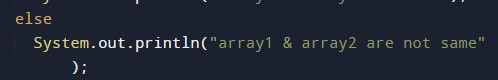In this tutorial we have learn about the Java Program to Compare Two Arrays and its application with practical example. I hope you will like this tutorial.